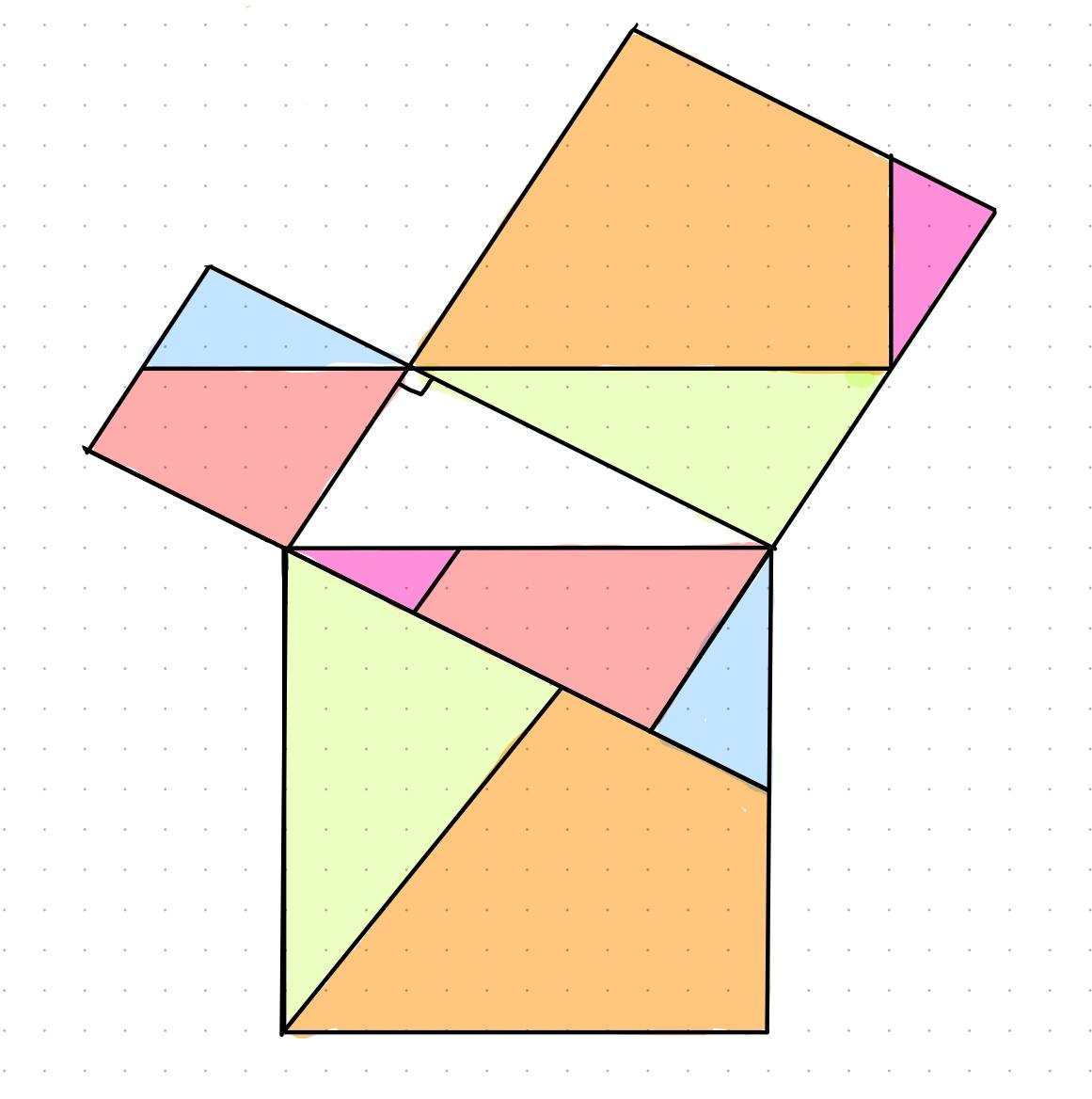# Deducing the Pythagorean Theorem from a particular dissection How can IAudrina Jackson 2022-07-01 Answered
Deducing the Pythagorean Theorem from a particular dissectionHow can I deduce the pythagorean theorem from the follow image?
I have been draw some parallels and I got the figure but I don't know how to deduct, some hint?
You can still ask an expert for help

• Live experts 24/7
• Questions are typically answered in as fast as 30 minutes
• Personalized clear answers

Solve your problem for the price of one coffee

• Math expert for every subject
• Pay only if we can solve itbillyfcash5n
Note that each colored piece in the big square with side length c appears once in the small squares with side lengths a and b. From this, the sum of the areas of the small squares is equal to the area of the big square, or $\overline{){a}^{2}+{b}^{2}={c}^{2}}$

We have step-by-step solutions for your answer!